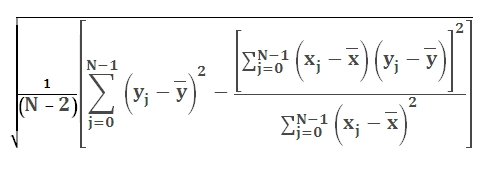# SQL Server STEYX function

Updated: 9 August 2010

Use STEYX_q to return the standard error of the predicted y-value for each x-value in the regression. The equation for STEYX_q is:Syntax
SELECT [wctStatistics].[wct].[STEYX_q] (
<@Known_y_Known_x_RangeQuery, nvarchar(4000),>)
Arguments
@Known_y_Known_x_RangeQuery
the select statement, as text, used to determine the y- and x-values to be used in the STEYX_q function.
Return Types
float
Remarks
·         STEYX_q assumes that its arguments are the entire population. If your data represent a sample of the population, then compute the standard deviation using STDEV_q.
·         For large samples sizes, STDEV_q and STEYX_q return approximately equal values.
·         No GROUP BY is required for this function even though it produces aggregated results.
Examples

To determine standard error between grades in math and grades in science:
from #s1 a, #s1 b
where a.subject = ' + Char(39) + 'Math' + Char(39) + ' and
b.subject = ' + Char(39) + 'Science' + Char(39) + 'and
a.student = b.student')

This produces the following result

----------------------
0.00426728110387755

(1 row(s) affected)

To determine the correlation between grades in math and grades in science on a de-normalized table:

SELECT wct.STEYX_q('SELECT math, science from #s2')

This produces the following result

----------------------
0.00426728110387755

(1 row(s) affected)

### SupportCopyright 2008-2021 Westclintech LLC         Privacy Policy        Terms of Service